# Terminology

In this talk, we will be concerned with Art and Illusion as Gombrick described it. For the moment, however, I would like to keep it simple and switch to a real pipe like this one in my hand. Later, when we have a functional terminology, we can take on the extra layers of complexity associated with the fact that the projector is actually presenting a computer generated image, of a photograph, of a postcard, of a painting by Magritte, of an object we call a pipe.

When you look in my direction, you see a visual Image that your visual system has constructed of an external scene, and part of the scene contains a pattern of light reflected by a real object. The pattern of light produces a Pattern of neural activity in your visual system and you see the Pattern (of the pattern) as a Figure because it is an organized subsection of the visual Image. The Figure appears to represent an object in the external world -- an object which becomes a visual Object when it is recognized as a pipe, or some other significant properties are attached to it. These concepts and their relationships might be represented by the expression below. To make it clear at all times, what is in the external world and what is inside the head, things inside the head are capitalized and things in the external world are not.$\mathrm{Image} \bigl[ \mathrm{Object} \bigl( \mathrm{Figure} ( \mathrm{Pattern(object)}) \bigr) \bigr] \quad\xleftarrow{\quad V \quad}\quad \textrm{scene}\bigl[ \mathrm{pattern(object)} \bigr]$

The arrow with the V over it means 'is converted by the visual system into'. Using the obvious abbreviations, a simple notation for this expression, describing the relationship between the external scene with its object and the corresponding internal representations, might be$I \bigl[ O \bigl( F (P(o) ) \bigr) \bigr] \quad\xleftarrow{\quad V \quad}\quad s \bigl[ p(o) \bigr]$

With this notation, we are almost ready to tackle the representation of auditory objects; however, we need one more concept. All sound and auditory experience exists over time by its nature; and so we need to extend the visual part of the analogy to include external events and visual Events before considering the analogy with the acoustic world and hearing. If I pick up my pipe and raise it to my mouth to create an event, you see the pipe Object rise and rotate over time. The external event can be represented, using animation, as a sequence of patterns of light, in which case the visual Event is a sequence of visual Figures constructed from a sequence of neural Patterns arising in response to a sequence of patterns of light from an external object. In functional form, the initial expression becomes,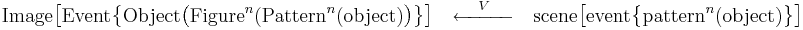$\mathrm{Image} \bigl[ \mathrm{Event} \bigl\{ \mathrm{Object} \bigl( \mathrm{Figure}^n ( \mathrm{Pattern}^n (\mathrm{object}) \bigr) \bigr\} \bigr] \quad\xleftarrow{\quad V \quad}\quad \mathrm{scene} \bigl[ \mathrm{event} \bigl\{ \mathrm{pattern}^n ( \mathrm{object} ) \bigr\} \bigr]$

where$\ o =$ external object, and ‘sequence of’ is noted by braces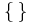$\{\,\}$ and a superscript index. Thus, the expression,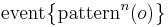$\mathrm{event} \bigl\{ \mathrm{pattern}^n(o)\bigr\}$, means ‘an event, which is a sequence of n patterns of light from an object’. The notation for the expression is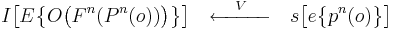$I \bigl[ E \bigl\{ O \bigl( F^n(P^n(o)) \bigr) \bigr\} \bigr] \quad\xleftarrow{\quad V \quad}\quad s \bigl[ e \bigl\{ p^n(o) \bigr\} \bigr]$

and it might be read as follows:

‘You experience a visual Image,$I [\ \cdot\ ]$, containing a visual Event,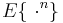$E \{\ \cdot^n \}$ , composed of a visual Object,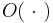$O(\ \cdot\ )$, moving. The visual Object is a sequence of visual Figure frames,$F \{\ \cdot^n \}$, corresponding to a sequence of neural Patterns,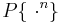$P \{\ \cdot^n \}$, of the external object. Your visual system constructed your visual experience,$\xleftarrow{\ V \ }$ , from an external scene,$s [\ \cdot\ ]$, containing an optical event,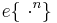$e\{\ \cdot^n \}$, which is a sequence of patterns of reflected light of a real pipe,$p_r^n(o)$.'

Finally, before proceeding to auditory Objects and Events, we need to provide the notation with the ability to express two more simple distinctions; firstly, the distinction between Visual (V) and Auditory (A), with the corresponding external distinction between optical (o) and acoustical (a), and secondly, the distinction between reflected energy (r) and emitted energy (e). Visual Objects and Events are more commonly associated with light that is reflected from an object -- light which comes from a source that is not the immediate concern of the discussion of perception, whereas auditory Objects and Events are more commonly associated with sounds that are directly emitted by the source of the perceptions under discussion, or to be more specific, by the resonators in the source. (It is this probably the distinction between reflected light and emitted sounds that prompts people to refer more to sources in auditory perception, as in 'sound sources', rather than to acoustic objects.)

The distinctions described in the previous paragraph will be made, in the usual way, by attaching subscripts to the variables. So to distinguish the visual part of the part of the perception described above from any accompanying auditory, tactile or olfactory part of the Object or Event, the expression should be amended to read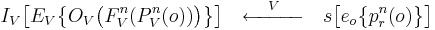$I_V \bigl[ E_V \bigl\{ O_V\bigl( F_V^n(P_V^n(o)) \bigr) \bigr\} \bigr] \quad\xleftarrow{\quad V \quad}\quad s \bigl[ e_o \bigl\{ p_r^n(o) \bigr\} \bigr]$

Here, then, is a compact, reasonably comprehensive notation that can express the basic relationships between external objects and events, on the one hand, and between the internal, mental representations of these objects and events on the other, and do so at a sequence of processing levels, of the sort that need to be distinguished in discussions of perception, to a greater or lesser degree. The notation has been developed using the visual example, not only because it is familiar, but also to make clear the level of complexity that is required to talk about perception in any modality, and so that the reader does not get the impression that the complexity of the notation is associated with auditory perception per se.

Note that we are using the word 'sound' (as with 'object' and 'event') to refer to acoustic waves in the external world as well as what we hear in response to the acoustic wave. (So for purposes of the discussion of auditory objects, the answer to the question, 'If a conifer falls in the woods and there is no one around to hear it, is there a sound?' is 'Yes'.)